# Multi-State Models for Oncology

library(oncomsm)
library(dplyr, warn.conflicts = FALSE)
library(ggplot2)

tl;dr: The multi-state characteristics of RECIST-like visit data in oncology can be exploited to reduce bias at interim analyses of objective response rate and drive event prediction for probability of success/go calculations.

In early oncology trials, (objective tumor) response based on the RECIST criteria is often used as primary endpoint to establish the activity of a treatment. Often, response is treated as binary variable although it is a delayed event endpoint. At the final analysis, this simplification is of little concern since all individuals tend to be followed up long enough to ignore the small amount of censoring. However, when continuously monitoring such a trial, the assumption of sufficient follow-up is no longer fulfilled at interim analyses and a simple binary analysis is biased.

The problem can be addressed by extending the statistic binary response model to a three-state model for “stable”, “response”, and “progression or death”. The respective transition numbers are given in the graph below.

Often, a hazard-based approach is used to model multi-state data. However, a hazard-based approach has several disadvantages:

1. The hazard scale is difficult to interpret since it is a momentary risk, not a probability. This leads to problems with prior specification in a Bayesian setting. The Bayesian approach is, however, particularly useful in the early development process since it allows to augment data with prior opinion or evidence and thus improve accuracy.

2. A hazard based, non-Markov multi-state model leads to intractable expressions for the implicitly given transition probabilities. Hence they need to be calculated by simulation which makes the model less convenient to work with if transition probabilities a or of primary interest. Since the (objective) response rate often plays an important role in the analysis of early oncology trials, this is a disadvantage.

An alternative framework to model multi-state data are mixture models. For details, see Jackson et al. (2022) and Jackson (2022). Here, we describe the concrete application to he simplified “stable”, “response”, “progression” model. The approach is similar to Aubel et al. (2021) and Beyer et al. (2020).

Here, a semi-Markov approach is used. This means that the time to the next transition only depends on the time already spent in a state, not on the full history of previous jumps. Additionally, it is assumed that the transition times between states conditional on both originating and target state can be described by Weibull distributions. This parametric family encompasses the exponential distribution with constant transition rates as special cases but also allows increasing or decreasing hazards over time.

Let $$T_{S}$$ be the transition time from the “stable” state and $$T_{R}$$ the transition time from the “response” state (also “sojourn” times). Let further $$R$$ be a binary random variable with $$R=1$$ if a response occurs, then the model can be specified as:

\begin{align} R &\sim \operatorname{Bernoulli}(p) \\ T_{S} \,|\, R = 1 &\sim \operatorname{Weibull}(k_1, \lambda_1) \\ T_{S} \,|\, R = 0 &\sim \operatorname{Weibull}(k_2, \lambda_2) \\ T_{R} &\sim \operatorname{Weibull}(k_3, \lambda_3) \end{align} where $$k$$ is the vector of shape- and $$\lambda$$ the vector of scale parameters. Let further $$f_i(t)$$ be the PDF of the Weibull distribution of transition $$i\in\{1,2,3\}$$ as indicated in the above figure and let $$F_i(t)$$ the corresponding CDF. This model implies that $\operatorname{Pr}\big[\,T_{S} > t \,\big] = p\cdot(1 - F_1(t)) + (1 - p)\cdot(1 - F_2(t))\\$ hence, it can be seen as a mixture model.

The median of a Weibull-distributed random variable is directly related to shape and scale parameters. Since the former is more convenient to interpret, the Weibull distributions are parameterized directly via their shape and median. The scale parameter can then be recovered via the relationship

$\operatorname{scale} = \frac{\operatorname{median}}{\log(2)^{1/\operatorname{shape}}} .$

The following prior classes are used.

For the response probability $$p$$, a $$(1-\eta)\,\operatorname{Beta}(a,b)+\eta\,\operatorname{Unif}(0,1)$$ is used. The prior is specified via the equivalent sample size $$a+b$$ and the mean of the informative component $$a/(a+b)$$.

A log-normal distribution is used for the median time-to-next event, the parameters are inferred from specified $$0.05$$ and $$0.95$$ quantiles.

A log-normal distribution is used for the shape parameter of the Weibull distributions, the parameters are inferred from specified $$0.05$$ and $$0.95$$ quantiles.

It is assumed that the observation process (visit spacing) is fixed. Since transitions can only be recorded after the next visit, all transition times are interval censored.

Recruitment times are assumed to follow a poisson distribution.

Default parameters assume a timescale in months.

## Specifying the model

The following code defined the prior assumptions for a two-group trial with a visit-spacing of 1 months. The prior variance on the shape parameter is very low. For sampling from the prior, this does not not matter and more uncertainty about the Weibull shape parameters can be assumed. During posterior inference, identifying shape and scale (median time to event) from a small sample of interval censored observations is not feasible and leads to divergent MCMC samples. The shape thus has to be kept almost fixed in most cases when doing inference.

mdl <- create_srpmodel(
A = define_srp_prior(
p_mean = 0.4, p_n = 10,
median_t_q05 = c(3, 2, 6) - 1, # s->r s->p r->p
median_t_q95 = c(3, 2, 6) + 1
),
B = define_srp_prior(
p_mean = 0.6, p_n = 10,
median_t_q05 = c(2, 8, 3) - 1, # s->r s->p r->p
median_t_q95 = c(2, 8, 12) + 1,
shape_q05 = c(2, 2, 0.75),
shape_q95 = c(2.1, 2.1, 0.76)
)
)

print(mdl)
#> srpmodel<A,B>

The model assumptions can be visualized by sampling from the prior.

## Prior checks

First, we plot the cumulative distribution functions (CDF) of the time-to-next-event over the first 36 (months) and the CDF of the response probabilities per group. These are based on a sample drawn from the prior distribution of the model. We can re-use the same parameter sample for sampling from the prior-predictive distribution by separating the sampling from the plotting steps.

Often, the rate of progression free survival (PFS) at a particular time point is of interest. This quantity is a direct function of the model parameters. Since the simplified model does not distinguish between progression or death, we denote the combined endpoint as “progression”. \begin{align} \operatorname{PFS}(t) :&= \operatorname{Pr}\big[\,\text{no progression before } t\,\big] \\ &= 1 - \operatorname{Pr}\big[\,\text{progression before } t\,] \\ &= 1 - \Big(\ \operatorname{Pr}\big[\,\text{progression before } t\,|\, \text{response}\,]\cdot\operatorname{Pr}\big[\,\text{response}\,] \\ &\qquad+ \operatorname{Pr}\big[\,\text{progression before } t\,|\, \text{no response}\,]\cdot\operatorname{Pr}\big[\,\text{no response}\,] \ \Big)\\ &= 1 - p\cdot\int_0^t f_1(u) \cdot F_3(t - u) \operatorname{d}u - (1 - p)\cdot F_2(t) \ . \end{align} The integral arises from the need to reflect the uncertainty over the state change from “stable” to “response” on the way to “progression”. Any parameter sample thus also induces a sample of the PFS rate at any given time point and the curve of PFS rate over time corresponds to the survival function of the “progression or death” event.

smpl_prior <- sample_prior(mdl, seed = 36L)

# plot(mdl) also works but need to resample prior further below
plot(mdl, parameter_sample = smpl_prior, confidence = 0.75)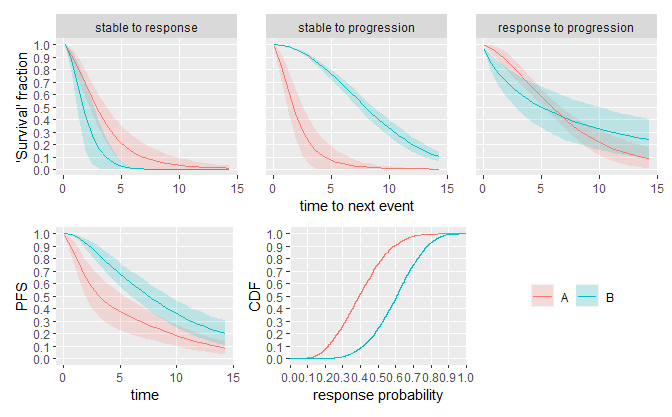## Sampling from the prior-predictive distribution

Next, we draw samples from the prior-predictive distribution of the model. We sample 100 trials with 30 individuals per arm. Here, we can re-use the sample prior sample already used for plotting.

tbl_prior_predictive <- sample_predictive(
mdl,
sample = smpl_prior,
n_per_group = c(30L, 30L),
nsim = 100,
seed = 342
)

print(tbl_prior_predictive, n = 25)
#> # A tibble: 58,631 × 5
#>    subject_id group_id     t state        iter
#>    <chr>      <chr>    <dbl> <chr>       <int>
#>  1 ID00010865 B         11.2 stable          1
#>  2 ID00010865 B         12.2 stable          1
#>  3 ID00010865 B         13.2 response        1
#>  4 ID00010865 B         14.2 response        1
#>  5 ID00010865 B         15.2 response        1
#>  6 ID00010865 B         16.2 response        1
#>  7 ID00010865 B         17.2 response        1
#>  8 ID00010865 B         18.2 response        1
#>  9 ID00010865 B         19.2 response        1
#> 10 ID00010865 B         20.2 response        1
#> 11 ID00010865 B         21.2 response        1
#> 12 ID00010865 B         22.2 response        1
#> 13 ID00010865 B         23.2 response        1
#> 14 ID00010865 B         24.2 response        1
#> 15 ID00010865 B         25.2 response        1
#> 16 ID00010865 B         26.2 response        1
#> 17 ID00010865 B         27.2 response        1
#> 18 ID00010865 B         28.2 response        1
#> 19 ID00010865 B         29.2 response        1
#> 20 ID00010865 B         30.2 progression     1
#> 21 ID00019694 B         18.2 stable          1
#> 22 ID00019694 B         19.2 stable          1
#> 23 ID00019694 B         20.2 stable          1
#> 24 ID00019694 B         21.2 stable          1
#> 25 ID00019694 B         22.2 stable          1
#> # ℹ 58,606 more rows

We can then run some quick checks on the sampled data, e.g., the observed response rates.

tbl_prior_predictive %>%
group_by(group_id, iter, subject_id) %>%
summarize(
responder = any(state == "response"),
.groups = "drop"
) %>%
group_by(group_id) %>%
summarize(
p_response = mean(responder),
se = sd(responder) / sqrt(n())
)
#> # A tibble: 2 × 3
#>   group_id p_response      se
#>   <chr>         <dbl>   <dbl>
#> 1 A             0.384 0.00888
#> 2 B             0.513 0.00913

A crude approximation of the median transition times can be compared with the prior means.

tbl_prior_predictive %>%
distinct(subject_id, iter, state, .keep_all = TRUE) %>%
group_by(iter, group_id, subject_id) %>%
summarize(
dt = t - lag(t),
from = lag(state),
to = state,
.groups = "drop"
) %>%
filter(to != "stable") %>%
group_by(group_id, from, to) %>%
summarize(
median transition time = median(dt),
.groups = "drop"
)
#> Warning: Returning more (or less) than 1 row per summarise() group was deprecated in
#> dplyr 1.1.0.
#> ℹ Please use reframe() instead.
#> ℹ When switching from summarise() to reframe(), remember that reframe()
#>   always returns an ungrouped data frame and adjust accordingly.
#> Call lifecycle::last_lifecycle_warnings() to see where this warning was
#> generated.
#> # A tibble: 6 × 4
#>   group_id from     to          median transition time
#>   <chr>    <chr>    <chr>                          <dbl>
#> 1 A        response progression                        6
#> 2 A        stable   progression                        2
#> 3 A        stable   response                           3
#> 4 B        response progression                        6
#> 5 B        stable   progression                        7
#> 6 B        stable   response                           2

By default, the prior predictive distribution is given in terms of panel visit data. The data can be transformed to interval-censored multi-state representation, (here only first sampled trial).

tbl_mstate <- tbl_prior_predictive %>%
filter(iter == 1) %>%
visits_to_mstate(mdl)

tbl_mstate
#> # A tibble: 84 × 7
#>    subject_id group_id from     to          t_min t_max t_sot
#>    <chr>      <chr>    <chr>    <chr>       <dbl> <dbl> <dbl>
#>  1 ID00010865 B        stable   response    12.2  13.2  11.2
#>  2 ID00010865 B        response progression 29.2  30.2  11.2
#>  3 ID00019694 B        stable   progression 30.2  31.2  18.2
#>  4 ID00294641 B        stable   progression 29.8  30.8  23.8
#>  5 ID00559801 B        stable   response    22.9  23.9  22.9
#>  6 ID00559801 B        response progression 29.9  30.9  22.9
#>  7 ID00827488 A        stable   response    13.2  14.2   7.19
#>  8 ID00827488 A        response progression 15.2  16.2   7.19
#>  9 ID01007254 A        stable   progression 25.4  26.4  23.4
#> 10 ID01039842 A        stable   progression  2.45  3.45  1.45
#> # ℹ 74 more rows

The multi-state data can be visualized in swimmer plots.

plot_mstate(tbl_mstate, mdl)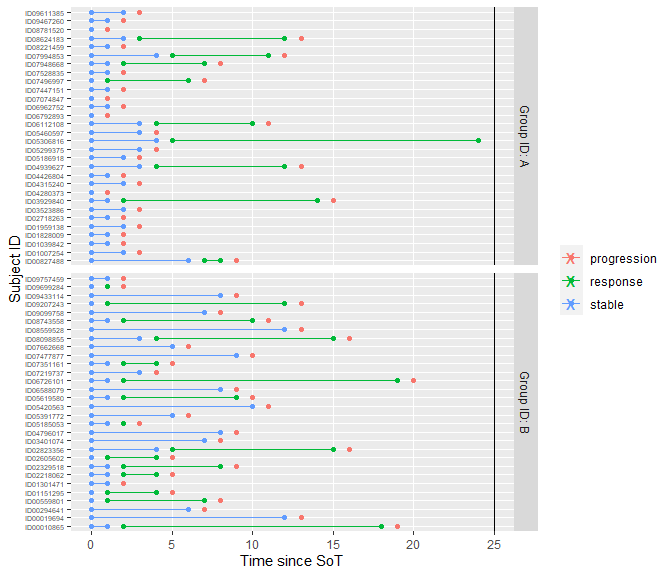It is also possible to simulate from the prior predictive distribution while fixing some of the parameter values. Fixing parameter values can be interpreted as conditioning on some or all of the parameters. For instance one could set the response probabilities to fixed values of $$0.1$$ and $$0.9$$:

sample_predictive(
mdl,
sample = smpl_prior,
p = c(0.1, 0.9),
n_per_group = c(30L, 30L),
nsim = 100,
seed = 3423423
) %>%
group_by(group_id, iter, subject_id) %>%
summarize(
responder = any(state == "response"),
.groups = "drop"
) %>%
group_by(group_id) %>%
summarize(
p_response = mean(responder),
se = sd(responder) / sqrt(n())
)
#> # A tibble: 2 × 3
#>   group_id p_response      se
#>   <chr>         <dbl>   <dbl>
#> 1 A             0.106 0.00561
#> 2 B             0.813 0.00712

## A hypothetical interim analysis

First, we sample a single data set under extreme response probabilities that deviate from the chosen prior. The data can then be curtailed to a hypothetical interim time-point simply by filtering the visit time-points.

tbl_data_interim <- sample_predictive(
mdl,
sample = smpl_prior,
p = c(0.2, 0.8),
n_per_group = c(30L, 30L),
nsim = 1,
seed = 42L
) %>%
filter(
t <= 15
)

The censoring in the interim data can be visualized in a swimmer plot again.

tbl_data_interim %>%
visits_to_mstate(mdl, now = 15) %>%
plot_mstate(mdl, relative_to_sot = FALSE, now = 15)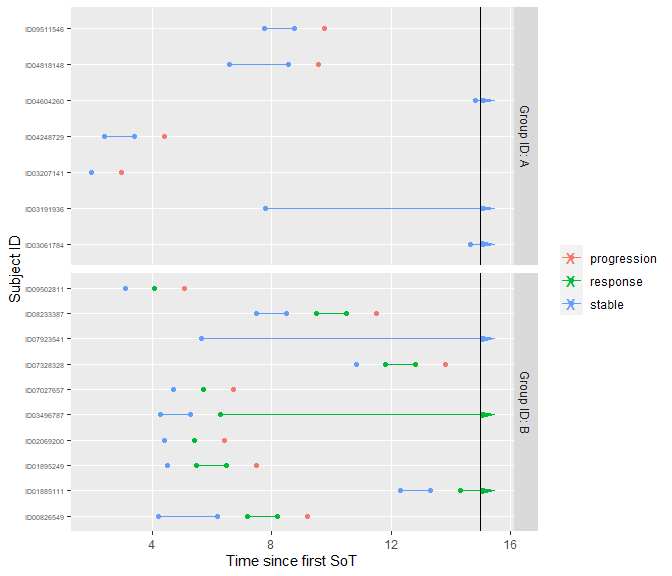We can check the observed response rates again. Due to censoring at the interim time point, the response rate estimate is biased.

tbl_data_interim %>%
group_by(group_id, iter, subject_id) %>%
summarize(
responder = any(state == "response"),
.groups = "drop"
) %>%
group_by(group_id) %>%
summarize(
p_response = mean(responder),
se = sd(responder) / sqrt(n())
)
#> # A tibble: 2 × 3
#>   group_id p_response    se
#>   <chr>         <dbl> <dbl>
#> 1 A               0     0
#> 2 B               0.9   0.1

Instead, one can now do inference by drawing sample from the posterior distribution this will account for censoring. Since the data conflicts with the prior, the posterior mass will move in the direction of the observed response rates.

smpl_posterior <- sample_posterior(mdl, tbl_data_interim, seed = 43L)
# plot under posterior
plot(mdl, parameter_sample = smpl_posterior, confidence = 0.75)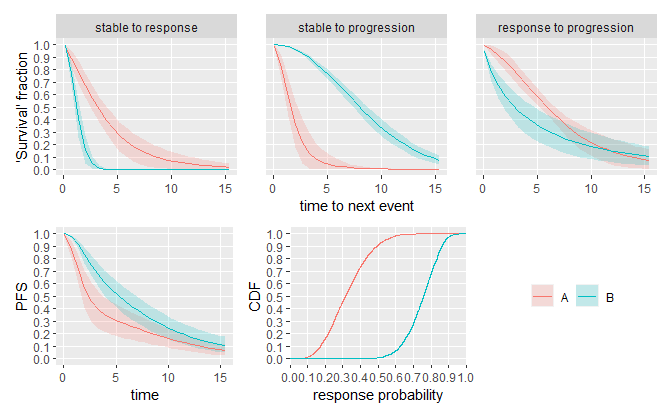# calculate posterior quantiles of response probability
smpl_posterior %>%
parameter_sample_to_tibble(mdl, .) %>%
filter(parameter == "p") %>%
group_by(group_id) %>%
summarize(
p_posterior_mean = median(value),
q25 = quantile(value, probs = .25),
q75 = quantile(value, probs = .75)
)
#> # A tibble: 2 × 4
#>   group_id p_posterior_mean   q25   q75
#>   <chr>               <dbl> <dbl> <dbl>
#> 1 A                   0.314 0.235 0.407
#> 2 B                   0.758 0.692 0.818

Alternatively, the analysis could also be run using the default weakly-informative prior. For details on the prior choice, see the corresponding vignette.

mdl2 <- create_srpmodel(
A = define_srp_prior(),
B = define_srp_prior()
)
smpl_posterior2 <- sample_posterior(mdl2, tbl_data_interim, seed = 43L)
# plot under posterior
plot(mdl2, parameter_sample = smpl_posterior2, confidence = 0.75)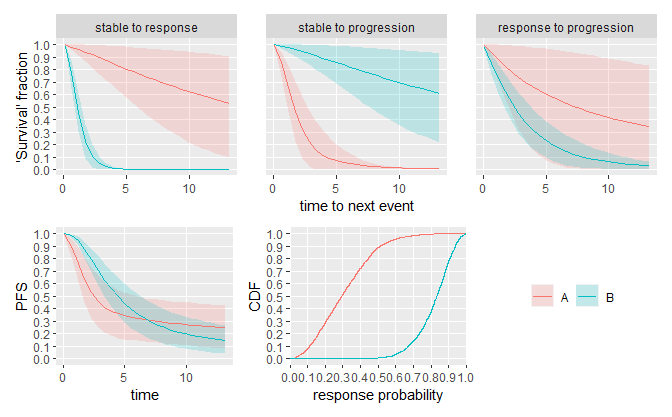# calculate posterior quantiles of response probability
smpl_posterior2 %>%
parameter_sample_to_tibble(mdl2, .) %>%
filter(parameter == "p") %>%
group_by(group_id) %>%
summarize(
p_posterior_mean = median(value),
q25 = quantile(value, probs = .25),
q75 = quantile(value, probs = .75)
)
#> # A tibble: 2 × 4
#>   group_id p_posterior_mean   q25   q75
#>   <chr>               <dbl> <dbl> <dbl>
#> 1 A                   0.295 0.183 0.415
#> 2 B                   0.833 0.756 0.892

## Session info

sessionInfo()
#> R version 4.2.2 (2022-10-31 ucrt)
#> Platform: x86_64-w64-mingw32/x64 (64-bit)
#> Running under: Windows 10 x64 (build 19044)
#>
#> Matrix products: default
#>
#> locale:
#>  LC_COLLATE=C
#>  LC_CTYPE=English_United States.utf8
#>  LC_MONETARY=English_United States.utf8
#>  LC_NUMERIC=C
#>  LC_TIME=English_United States.utf8
#>
#> attached base packages:
#>  stats     graphics  grDevices utils     datasets  methods   base
#>
#> other attached packages:
#>  ggplot2_3.4.1 oncomsm_0.1.4 tidyr_1.3.0   dplyr_1.1.1
#>
#> loaded via a namespace (and not attached):
#>   Rcpp_1.0.10          listenv_0.9.0        visNetwork_2.1.2
#>   prettyunits_1.1.1    ps_1.7.3             digest_0.6.31
#>   utf8_1.2.3           parallelly_1.34.0    R6_2.5.1
#>  backports_1.4.1      stats4_4.2.2         evaluate_0.20
#>  highr_0.10           pillar_1.9.0         rlang_1.1.0
#>  rstudioapi_0.14      furrr_0.3.1          callr_3.7.3
#>  jquerylib_0.1.4      checkmate_2.1.0      DiagrammeR_1.0.9
#>  rmarkdown_2.21       labeling_0.4.2       stringr_1.5.0
#>  htmlwidgets_1.6.2    loo_2.5.1            munsell_0.5.0
#>  compiler_4.2.2       xfun_0.38            rstan_2.21.8
#>  pkgconfig_2.0.3      pkgbuild_1.4.0       globals_0.16.2
#>  rstantools_2.3.0     htmltools_0.5.5      tidyselect_1.2.0
#>  tibble_3.2.0         gridExtra_2.3        codetools_0.2-18
#>  matrixStats_0.63.0   fansi_1.0.4          future_1.32.0
#>  crayon_1.5.2         withr_2.5.0          grid_4.2.2
#>  jsonlite_1.8.4       gtable_0.3.3         lifecycle_1.0.3
#>  patchwork_1.1.2      sass_0.4.5